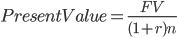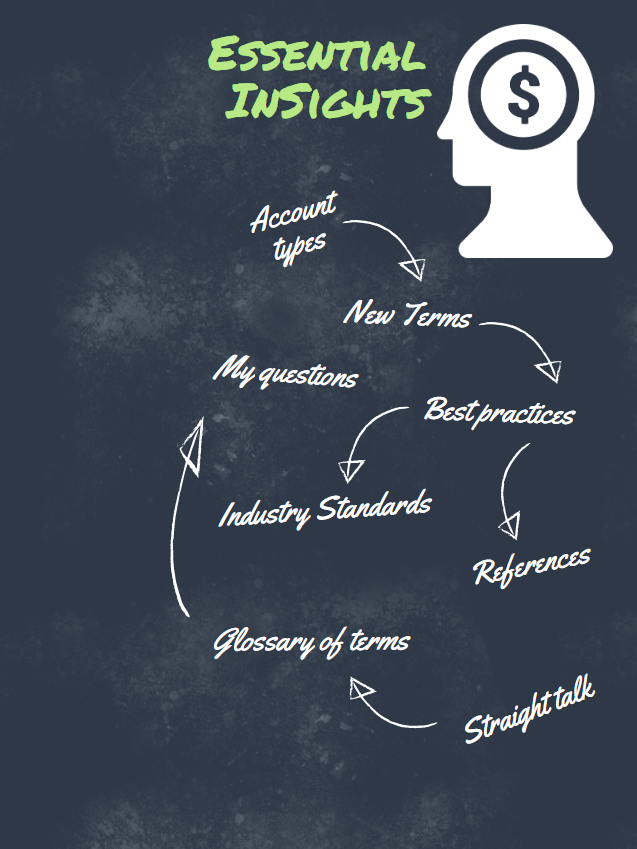InSight

# Definitions: Present Value (PV)

The present value (PV) is the current value of all future sums of money or stream of cash flows given a specified rate of return. Future cash flows are discounted based on the time value of money referred to here as the discount rate, and the higher the discount rate, the lower the present value of the future cash flows. So at it root it represents a standard way of valuing an investment in todays terms.

##$$Present Value = \frac{FV}{(1+r)n}$$## Other Related Topics:

### Definitions: Fixed Income

Fixed Income (or debt) represents your ownership over the repayment of a debt. Usually considered bonds, they are contracts promising the repayment of loaned money.

### Definitions: Equity

The term Equity represents any ownership rights over an asset’s cash flow generation potential. As an asset class, there is no guarantee of a return